# Determination of quantity supplied by firm in perfectly competitive market in the short run

(diff) ← Older revision | Latest revision (diff) | Newer revision → (diff)

This article describes the process by which a firm selling a good, service or commodity in a perfectly competitive market decides the quantity to be supplied.

The chief characteristics of a perfectly competitive market, that are relevant here, are as follows:

1. Each firm is small in size relative to the totality of all firms supplying the good, and is competing solely on price (i.e., with no product differentiation).
2. The firm is a price taker rather than a price setter, i.e., it has no leeway in deciding the price and must stick to the price prevailing in the market (there are exceptions to this when the price is not at the market equilibrium; see the convergence towards market price page).
3. Whatever quantity the firm produces within its natural limitations can be completely sold at the market, i.e., the firm does not have to worry about unsold inventory as long as it prices products at the market price.

Setting the price at the market price is not (necessarily) enforced through any legal regulation, but is forced for the following two reasons:

• Because the firm is very small relative to the whole market, reducing the price below the market price will shift much more consumer demand to that firm than the firm can handle.
• Similarly, increasing the price above the market price will cause the firm to lose all its customers to competing firms.

The overall conclusion will be the following:

The absolute (i.e., global) maximum for profit must occur either at one of these three points: zero quantity of production, the maximum quantity of production, or some local maximum at an intermediate quantity of production. In the last case, that must be a point where$P = MC$ (where$P$ is the price and$MC$ is the marginal cost of production) and the$MC$ curve is rising.

For the meat of the analysis, see the formal mathematical analysis section.

As an immediate corollary:

The short run supply curve (where the optimal quantity produced is plotted as a function of market price) coincides geometrically with (part of) the marginal cost curve (where marginal cost is plotted as a function of quantity produced).

Note that the analysis also applies to monopsony, where there is a single buyer, as long as there is perfect competition among sellers.

## Two interpretations of this determination

This determination can be interpreted in either of these ways:

1. The market price for the firm is known, and we are optimizing for the firm's quantity supplied.
2. We are determining the optimal functional relationship that describes the quantity supplied by the firm in terms of market price (i.e., the short run supply curve).

With interpretation (2), interpretation (1) simply becomes the case where we have to determine one point on the curve. However, since the price coordinate for that point can be arbitrary, both interpretations require equivalent tasks.

## Similar determinations

This article focuses on the determination in the short run, as opposed to the long run. Also, it focuses on firms in perfectly competitive markets. We briefly describe the significance of both these conditions.

### Contrast with monopolistic firm

Further information: Determination of quantity supplied by monopolistic firm in the short run

There are three key differences. The first two are related and the third follows from them:

Parameter Firm in perfectly competitive market Monopolistic firm
control over market price no control. The firm must accept the market price. significant control. The firm sets both its price and quantity of production.
guarantee of selling everything it produces guaranteed, as long as it prices at the market price. no guarantee. The firm needs to choose its price appropriately based on its estimate of the nature of demand and the quantity it produces.
nature of marginal revenue curve (marginal revenue plotted on the vertical axis as a function of quantity produced on the horizontal axis) flat horizontal curve with price coordinate equal to the market price it's complicated. Roughly speaking, it is related to the demand curve facing the firm (the relationship is complicated and is of a marginal-to-average kind).

Another way of putting these is that monopolistic firms have more power (in the sense of setting prices) but also more responsibility (in the sense of having to figure what price to set).

Most of the analysis here regarding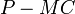$P - MC$ carries over to the monopolistic case, but the constant$P$ gets replaced by the variable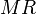$MR$ (marginal revenue) so the relevant difference is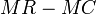$MR - MC$, and the$P = MC$ conditions get replaced by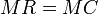$MR = MC$ conditions.

### Contrast with long run

The long run analysis differs from the short run analysis primarily in the following sense: the fixed costs are no longer fixed and can be decided based on the estimate of the quantity produced. There is also less of a hard constraint on the maximum quantity that can be produced.

Long run supply curves are more likely to be downward sloping under a wide range of circumstances. An industry with a downward sloping long run supply curve is termed a decreasing cost industry.

### Contrast with market as a whole

An individual firm simply determines the quantity supplied as a function of the price, rather than determining both. The market as a whole, however, determines both the total price and the quantity supplied, using two levels: the level of individual firms optimizing for the environment, and the level of market interactions between buyers and sellers.

## Cost and revenue definitions

We assume that the firm has two costs:

• Fixed costs are fixed in advance and cannot be altered in the short run. These may include investments in land or other fixed assets that cannot be acquired or got rid of quickly.
• Variable costs are costs that vary with the quantity produced.

We define:

Cost or revenue notion Acronym Units Definition How does it vary with quantity?
Fixed cost FC Money Cost of expenditure on fixed factors of production that cannot be varied in the short run Independent of quantity
Variable cost VC Money Cost of expenditure on factors of production that can be varied in the short run Increases with quantity
Total cost TC Money Fixed cost + Variable cost Increases with quantity
Average cost ATC, AC Money per unit quantity (Total cost)/Quantity Generally, first decreases and then increases with quantity
Average fixed cost AFC Money per unit quantity (Fixed cost)/Quantity Decreases with quantity (by definition, because denominator is increasing and numerator is constant).
Average variable cost AVC Money per unit quantity (Variable cost)/Quantity Generally, increases with quantity when quantity is large enough. For small quantities, it may decrease with quantity.
Marginal cost MC Money per unit quantity Derivative of total cost with respect to quantity; since fixed cost is independent of quantity, this is the same as the derivative of variable cost with respect to quantity Generally, increases with quantity when quantity is large enough. For small quantities, it may decrease with quantity.
Market price P Money per unit quantity The unit price in the market. Not under the control of the firm. Independent of quantity
Revenue R Money Revenue earned by producing a given quantity of the commodity. It equals the product of quantity produced and the (unit) market price. Note that because of the small size of the firm relative to the market, it must sell everything it produces at the market price Increases with quantity (by definition).
Marginal revenue MR Money per unit quantity Equals the market price P Independent of quantity
Profit (not accounting for fixed costs)  ? Money Equals R - VC, same as Q(P - AVC) where Q is the quantity produced, P is the unit price, and AVC is the average variable cost at the given value of Q. See discussion below
Profit (accounting for fixed costs)  ? Money Equals R - TC, same as Q(P - ATC), where Q is the quantity produced, P is the unit price, and AC is the average (total of fixed and variable) cost. In the short run, the firm may choose to produce even if the profit, after accounting for fixed costs, is negative. See discussion below

## Calculation problem for the firm

### Information available to the firm and its graphical representation

The firm has two pieces of information:

Piece of information Nature Equivalent pieces of information How it's decided
the marginal cost curve of the firm This is a curve describing the marginal cost as a function of quantity produced. Traditionally, the quantity is plotted along the horizontal axis and the cost function (in units money per unit quantity) along the vertical axis. It is not a single number but rather a functional relationship. Knowledge of the marginal cost curve is mathematically equivalent to knowledge of the average variable cost curve, the variable cost curve, and (subject to knowledge of the fixed cost),the average fixed cost curve, the average total cost curve, and the total cost curve. This means that knowledge of any one of these curves can be used to deduce the others. Determined by the firm's production technology and factors of production. The curve itself is not subject to change.
the market price (which can be thought of as the marginal revenue curve of the firm) This is a single number describing the unit price (price per unit) of the good in the market. The graph plotting the market price as a constant function of quantity produced is the firm's marginal revenue curve. This can be overlaid on the marginal cost curve. Determined by market-wide supply and demand. See market price for more.

### Goal of the firm

The firm's goal is to use the information available to it (its marginal cost curve and the market price) to choose a quantity to produce so as to maximize its profit (note that fixed costs are treated as sunk costs and thus do not affect the profit calculation). If the firm chooses to produce a quantity$Q$, the profit (the objective function to be maximized) (not accounting for fixed costs) is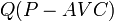$Q(P - AVC)$ where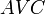$AVC$ is the average variable cost and is itself a function of$Q$. Note that accounting for fixed costs simply affects the profit by a constant additive factor and thus does not affect the choice of optimal quantity.

### Note on conventions for profit: accounting and not accounting for fixed costs

If$Q$ denotes the quantity produced,$P$ denotes the market price (which is also the same as the marginal and average revenue) then the total revenue is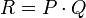$R = P \cdot Q$, and the total variable cost is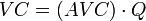$VC = (AVC) \cdot Q$ where$AVC$ is the average variable cost for the given choice of$Q$. The net profit (not accounting for fixed costs) is$R - VC$, which becomes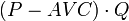$(P - AVC) \cdot Q$.

In particular, the net profit (not accounting for fixed costs) is zero at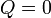$Q = 0$.

The net profit accounting for fixed costs is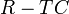$R - TC$, which becomes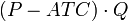$(P - ATC) \cdot Q$, where$AC$ is the average total cost, including both average fixed costs and average variable costs.

This is smaller than the profit calculated by not accounting for fixed costs. Also, for the short-run choices, this profit value may well be negative, because the firm treats its fixed costs as sunk costs.

The relationship between the two notions is:

Net profit accounting for fixed costs = Net profit not accounting for fixed costs - Fixed costs

In particular, the difference between the two (the fixed cost) is independent of quantity.

Note that as far as the maximization problem is concerned, both choices of the profit function are equivalent, because they differ by a value (fixed costs) that is constant (in the short run). However, there is an advantage to choosing the convention where we compute profits not accounting for fixed costs. The advantage is that it computes the profits relative to the situation of staying idle, i.e., producing nothing, and hence, can be more useful in quickly figuring out whether a certain quantity of production is more profitable than producing nothing.

Thus, for the purpose of our discussion, we use the term profit for the profit not accounting for fixed costs, given by$(P - AVC) \cdot Q$.

### Construction of the short run supply curve

The short run supply curve is constructed as follows: for each possible value of market price$P$, the firm solves the optimization problem above and computes the quantity to produce. This quantity to produce is then plotted as a function of market price, with price on the vertical axis and quantity on the horizontal axis.

It will turn out that the short run supply curve (where the optimal quantity produced is plotted as a function of market price) coincides geometrically with (part of) the marginal cost curve (where marginal cost is plotted as a function of quantity produced). This is not completely obvious but can be justified both formally and intuitively. We turn to this justification now.

## Formal mathematical analysis

If you find the formal analysis here intimidating, please read the next two sections and then return to the mathematical analysis if you wish.

### Determining the profit-maximizing quantity of production

We make the following assumptions:

• The firm can choose to produce any quantity between$0$ and a maximum quantity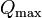$Q_{\max}$.
• The marginal cost$MC$ is defined at all quantities of production in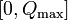$[0,Q_{\max}]$. Formally, it is the derivative of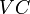$VC$ with respect to$Q$, though at the endpoints$0$ and$Q_{\max}$, we take one-sided derivatives.
• For simplicity, we also assume that the$MC$ curve is continuous and is not highly oscillatory, i.e., we can break up the interval$[0,Q_\max]$ into finitely many pieces and the curve is either increasing or decreasing on each piece.

Our goal is to prove the following:

The absolute (i.e., global) maximum for profit must occur either at one of these three points: zero quantity of production, the maximum quantity of production, or some local maximum at an intermediate quantity of production. In the last case, that must be a point where$P = MC$ and the$MC$ curve is rising.

We establish this formally in a few steps.

Step no. Assertion Explanation
1 The profit function (not accounting for fixed costs) is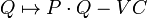$Q \mapsto P \cdot Q - VC$ where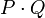$P \cdot Q$ is the product of the market price$P$ and the quantity produced$Q$. Profit (not accounting for fixed costs) is total revenue minus variable cost. Total revenue is the product$PQ$.
2 The derivative of the profit function with respect to$Q$ is$P - MC$ where$P$ is the market price and$MC$ is the marginal cost (dependent on$Q$). [SHOW MORE]
3 For those quantities of production where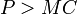$P > MC$, the profit function is increasing with respect to$Q$. For those quantities of production where$P < MC$, then the profit function is decreasing with respect to$Q$. See positive derivative implies increasing, negative derivative implies decreasing.
4 The local maxima for the profit function must occur at points where$P = MC$. Further, under reasonable assumptions of the function not being heavily oscillatory, we must have$P > MC$ for$Q$ slightly less than the point of local maximum and$P < MC$ for$Q$ slightly more than the point of local maximum. In other words, the$MC$ curve must be increasing and must cross the horizontal line for market price$P$ at the optimal quantity$Q$. Follows from (3). We can also interpret this in terms of the first derivative test applied to the profit function as a function of$Q$.
5 The absolute (i.e., global) maximum for profit must occur either at one of these three points: zero quantity of production, the maximum quantity of production, or some local maximum at an intermediate quantity of production. In the last case, that must be a point where$P = MC$ and the$MC$ curve is rising. Follows directly from Step (4).

### Putting the points together: constructing the curve

The goal of the above analysis was to find, for a given value of market price, the profit-maximizing quantity of production. Effectively, this helps plot one point in the short run supply curve. To construct the full short run supply curve, this analysis needs to be performed for every choice of market price.

Rather than do the full analysis, we make the following observation: for those choices of price where the quantity of production is not the extreme of$0$ or$Q_\max$, the (price,quantity) point pair that we obtain lies on the marginal cost curve. Thus, to oversimplify matters, (part of) the short run supply curve coincides with (part of) the marginal cost curve. Exactly what parts coincide depends on the precise nature of the curves. Two of the most common examples are discussed in the next section.

## Graphical interpretation of revenue and profit

We have the following:

• The revenue is given by the product of the price and quantity produced. It is the area of a rectangle with two sides along the axes and the optimal (quantity,price) vertex as the one vertex not on the axes.
• The profit is given by the signed area between the marginal cost curve and the horizontal line for the price, up till the optimal quantity produced. Explicitly, it is: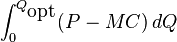$\int_0^{Q_{\mbox{opt}}} (P - MC) \, dQ$

where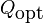$Q_{\mbox{opt}}$ is the optimal quantity.

## Important cases

Case Profit-maximizing choice Reservation price (minimum price for nonzero quantity produced) Short run supply curve Picture Details
The marginal cost is constant (in this case, the MC and AVC curves coincide and are horizontal) Produce at maximum quantity$Q_\max$ if the marginal cost is less than the market price, and produce nothing if it is greater than the market price. Constant value of marginal cost Let$\alpha$ be the constant value of marginal cost. Vertical line at quantity zero for price from 0 to$\alpha$, then jumps to vertical line at quantity$Q_\max$ from price$\alpha$ to infinite. Discontinuity at$\alpha$.
The marginal costs are increasing throughout, i.e., the marginal cost curve is upward sloping throughout. Profit-maximizing choice is unique quantity at which$P = MC$ (if it exists), otherwise 0 or$Q_\max$. Value of MC at quantity zero = value of AVC at quantity zero Short run supply curve coincides with marginal cost curve, plus vertical lines at ends.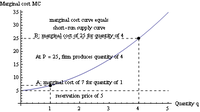Determination of quantity supplied by firm in perfectly competitive market in the short run with increasing marginal cost
The marginal costs are decreasing throughout, i.e., the marginal cost curve is downward sloping throughout. Produce either 0 or$Q_\max$, 0 if the price is less than the AVC at$Q_\max$, and$Q_\max$ if the price is greater than the AVC at$Q_\max$. value of AVC at quantity$Q_\max$ Let$\alpha$ be the value of AVC at$Q_\max$. Vertical line at quantity zero for price from 0 to$\alpha$, then jumps to vertical line at quantity$Q_\max$ from price$\alpha$ to infinite. Discontinuity at$\alpha$.
The marginal cost first decreases, then increases. Profit-maximizing choice is quantity at which$P = MC$ lying to the right of minimum AVC, otherwise 0 or$Q_\max$. Minimum AVC value Short run supply curve coincides with part of marginal cost curve to right of minimum AVC quantity (also called minimum efficient scale) plus vertical lines. It is discontinuous at minimum AVC.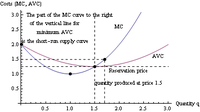Determination of quantity supplied by firm in perfectly competitive market in the short run with marginal cost that decreases then increases